# Solution assignment 03 Exponential functions and graphs

### Assignment 3

Draw the graph of the function: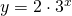Calculate the intersection point with the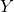-axis and the equation of the asymptote.

### Solution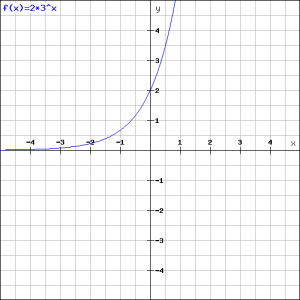The intersection point with the-axis can be found by substituting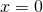in the function. Then we find: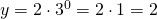The asymptote of the function is the line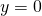(i.e. the-axis). The more negative, the more the graph approaches the-axis. We can verify this in the figure.

0